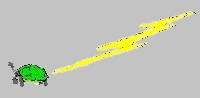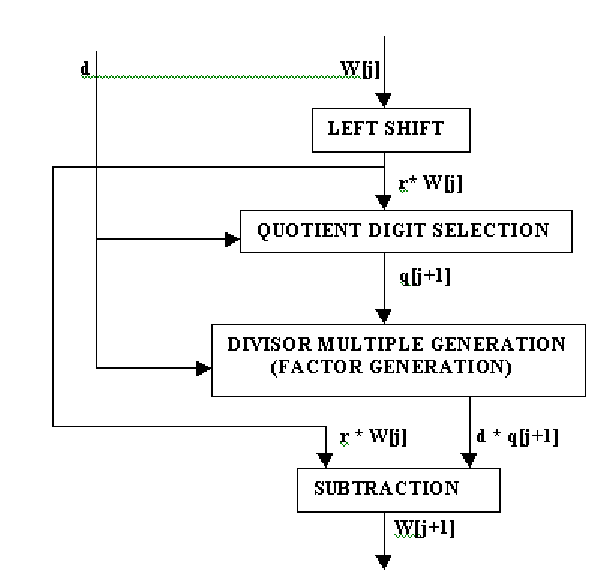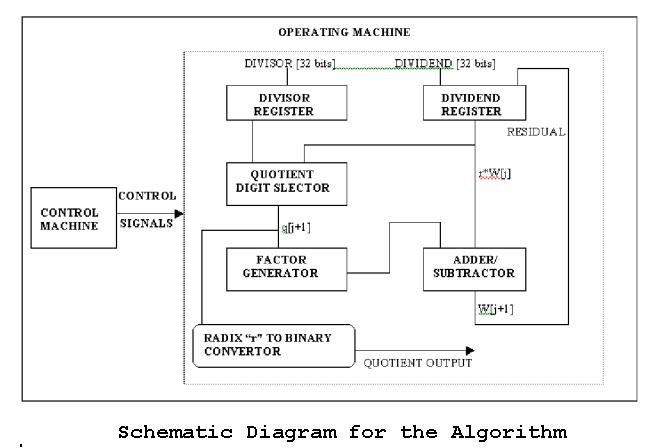# A scientific study of the problems of digital engineering for space flight systems, with a view to their practical solution.

Hardware Divider For Leon Processor

A European Space Agency have developed a processor named Leon. Synthesizable VHDL model of the processor is also made available. The project involves design and implementation of one of the following arithmetic units in two technologies; FPGA and Synopsys standard library.

Note: From http://www.cse.iitd.ernet.in/~vls00021/semester1/project/leon/algo.htm

Requirements

• Hardware divider. An iterative 1 bit/cycle signed and unsigned divider.
• The divider should do the following:
• Division performs integer division (signed & unsigned)
• Division by zero issues a warning (assertion)

Algorithm

The simplest form of algorithm for division is:

• A = B*Q + R
• Sign(R) = sign(A)
• Abs(R) < Abs(B)

This is called the Euclidian Division.

Various Algorithms For Division

The two main categories are

• The subtractive method:
• Subtractive algorithms are the most popular.
• They calculate the square root and quotient directly
• They can achieve low latencies with a relatively small amount of hardware
• Various algorithms for this method are:
• restoring
• non-restoring with non-redundant digit set and
• non-restoring with redundant digit set.

These methods are widely used in modern microprocessors and co-processors

• The multiplicative methods.
• Multiplicative algorithms do not calculate quotients and square roots directly but they use iterations to refine an approximation to the desired result. Moreover, they imply the use of a fast multiplier, generally very expensive in terms of silicon area.

The SRT non-restoring division algorithm
This algorithm corresponds to a recurrence where each iteration produces one redundant digit of the result.

The choice of the SRT algorithm is due to the following considerations:

• Many recent microprocessors use its implementations for both division and square root
• The architecture is sufficiently simple to be placed in a low cost programmable chip

The SRT method was discovered independently, at about the same time by Sweeny, Robertson and Tocher. Its digit-serial implementation represents a good compromise between computational speed and cost in term of silicon area. It is used in many floating-point arithmetic units of recent microprocessors. In the SRT algorithm, the quotient is computed as with the pencil and paper procedure. Thus, the following recurrence is used at every iteration:

W[j+1] = r*W[j] - d*q[j+1]

Where W[j] is the partial remainder at iteration j, q[j+1] is the (j+1)-th digit of the quotient, r is the radix, r*W=x is the dividend and d is the divisor.

The flowgraphThe control Machine operation:

1. The division block is in WAIT state to start with i.e. on processor reset. It comes out of the WAIT state on receiving the START signal from the control machine
2. On receiving the START signal the division block starts the operation only if the previous division operation is over i.e. only if it is in WAIT state. If the division block is busy then it indicates the same to the control machine & the control machine then keeps issuing the signal till the request is operated.
3. The dividend register is loaded with the dividend at the start of the first iteration. For the rest iterations it is loaded with the partial remainder/residual. The control machine issues signals to the operating machine to achieve this.

Project Team

Home
Last Revised: February 03, 2010
Digital Engineering Institute
Web Grunt: Richard Katz